Thermodynamics and Propulsion

# 16.2 Introduction to Conduction

We will start by examining conduction heat transfer. We must first determine how to relate the heat transfer to other properties (either mechanical, thermal, or geometrical). The answer to this is rooted in experiment, but it can be motivated by considering heat flow along a bar'' between two heat reservoirs at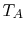,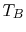as shown in Figure 16.2. It is plausible that the heat transfer rate,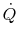, is a function of the temperature of the two reservoirs, the bar geometry and the bar properties. (Are there other factors that should be considered? If so, what?). This can be expressed as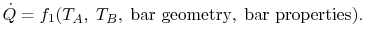(16..1)

It also seems reasonable to postulate thatshould depend on the temperature difference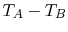. Ifis zero, then the heat transfer should also be zero. The temperature dependence can therefore be expressed as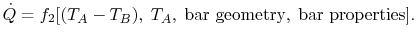(16..2)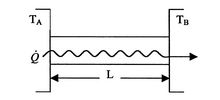An argument for the general form of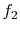can be made from physical considerations. One requirement, as said, is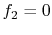if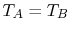. Using a MacLaurin series expansion, as follows,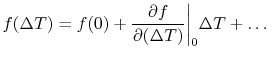(16..3)

If we define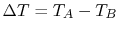and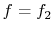, we find that (for small),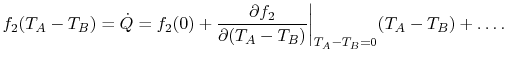(16..4)

We know that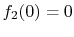. The derivative evaluated at(thermal equilibrium) is a measurable property of the bar. In addition, we know that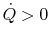if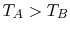or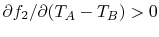. It also seems reasonable that if we had two bars of the same area, we would have twice the heat transfer, so that we can postulate thatis proportional to the area. Finally, although the argument is by no means rigorous, experience leads us to believe that as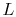increasesshould get smaller. All of these lead to the generalization (made by Fourier in 1807) that, for the bar, the derivative in Equation (16.4) has the form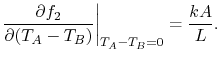(16..5)

In Equation (16.5),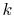is a proportionality factor that is a function of the material and the temperature,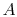is the cross-sectional area andis the length of the bar. In the limit for any temperature differenceacross a length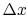as both,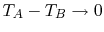, we can say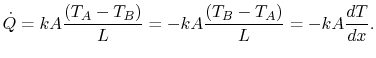(16..6)

A more useful quantity to work with is the heat transfer per unit area, defined as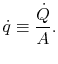(16..7)

The quantityis called the heat flux and its units are Watts/m2. The expression in (16.6) can be written in terms of heat flux as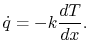(16..8)

Equation (16.8) is the one-dimensional form of Fourier's law of heat conduction. The proportionality constantis called the thermal conductivity. Its units are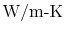. Thermal conductivity is a well-tabulated property for a large number of materials. Some values for familiar materials are given in Table 16.1; others can be found in the references. The thermal conductivity is a function of temperature and the values shown in Table 16.1 are for room temperature.

Table 16.1: Thermal conductivity at room temperature for some metals and non-metals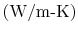Metals Ag Cu Al Fe Steel 420 390 200 70 50 Non-metalsAir Engine oilBrick Wood Cork 0.6 0.026 0.15 0.18 0.4-0.5 0.2 0.04

UnifiedTP